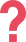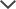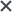Worth it. My son was taking the CogAT Level 5/6 for school and with help from the practice tests, he scored well and was able to get into the county's gifted program. Thank you so much.
Oliver
We got the Family Membership subscription to help our children become confident and familiar with the CogAT Level 10 test. We chose TestPrep-Online as it was a straight-forward site and felt trustworthy. The children said the tests were of very similar format and good fun. Totally recommended!
Mom of 2
The CogAT test pack provided my son with challenging questions, which prepared him to perform well on the test.
Subra
We used the NYC G&T pack. We ordered the materials a week before the test. My son was very happy and felt confident. Thanks!
Malak
We got the OLSAT/NNAT Bundle. We really like that we can watch the video academy multiple times. The site is easy and comfortable to use and I would recommend it to other parents of gifted children.
Anonymous
I used the Wonderlic personality prep pack. Your preparatory questions tuned my mind in a correct way to face the real questions.
Josh

## SSAT Elementary Level - Quantitative

The SSAT Elementary Level Quantitative section is made up of 30 questions to be completed in 30 minutes. These questions are based on concepts considered to be the fundamentals of 3rd and 4th grade mathematics. However, some of these questions may still be challenging for students in each respective grade. The questions on the SSAT math section for the Lower Level reflect the following:

Basic addition, subtraction, multiplication, and division Basic addition, subtraction, multiplication, and division
Place value Place value
Ordering of numbers (greater than, less than) Ordering of numbers (greater than, less than)
Fractions Fractions
Basic concepts of geometry (shapes and their attributes) Basic concepts of geometry (shapes and their attributes)
Basic concepts of measurement Basic concepts of measurement
Interpretation of graphs Interpretation of graphs
Factors and multiples
Patterns
Basic concepts of angle measurement

## SSAT Middle & Upper Levels - Quantitative

The math section on the SSAT Middle & Upper Levels is comprised of 50 questions, split into two parts (25 questions and 30 minutes each). These sections are used to assess one’s understanding and grasp of geometry and algebra, as well as other quantitative concepts. Many of the SSAT math problems are written in mathematical terms which directly indicate the operation necessary to resolve the question. Other questions are formatted as word problems which do not necessarily indicate the operation needed to solve the question. For such questions, one must examine the wording of the question and the information provided in order to determine what operations are necessary to solve the question.

The following table depicts the areas covered on the SSAT Middle & Upper Levels Quantitative sections:

Number Concepts and Operations Algebra (Elementary Concepts of Algebra) Geometry/ Measurement Data Analysis/ Probability
Arithmetic word problems (including percent, ratio) Properties of exponents Area and circumference of a circle Interpretation (tables, graphs)
Basic concepts of addition, subtraction, multiplication, and division Algebraic word problems Area and perimeter of a polygon Trends and inferences
Estimation Equations of lines Volume of a cube, cylinder, box Probability
Rational numbers Patterns Pythagorean theory and properties of right, isosceles, equilateral triangles
Sequences and series Absolute value Properties of parallel and perpendicular lines
Frequencies   Coordinate geometry
Slope

## SSAT Practice Test

Help your child succeed on the SSAT test! Make sure to set aside time to prepare with TestPrep-Online. We currently offer child-friendly upper SSAT practice tests and soon we will offer packs for the other two levels, to help your child succeed on the test. The SSAT prep packs include realistic practice tests and helpful study guides, allowing your child to become familiar with the test structure. Your child can start preparing for the SSAT test with TestPrep-Online today!

Some concepts in math come easily to students by practicing a lot. For example, operating with decimals becomes very intuitive after a few examples. Once you have added or subtracted fractions with unequal denominators you understand precisely what you need to do in general and can remember the rule. However, other topics like area, perimeter, volume, and others, require you to remember formulas and apply reasoning.

Probability is at first a difficult subject to learn, understanding the key formula to use, the differences between experiments, and events happening or not is something that also requires time. Information can also be displayed in different ways, by means of a bar graph, pie chart, pictograph, histogram, and more. All of these can express information differently and you should be able to draw conclusions from each form so dedicating some time into understanding these is also crucial for succeeding in the test.

Sequences and series requires imagination and a good control of algebraic skills. Even though there are formulas to calculate differences, random terms of a sequence, a sum, or others, having full control of this topic also demands a lot of practice. This is not a topic you want to skip or focus less.

In general, it is best if you summarize the information learned from classes into different study notes and try solving the questions that you have using these notes. Things like dividing, rational numbers, sequences, and equations may become more trivial but are still necessary. Remember also to go over all the geometric properties of shapes, related theorems, also learn how to identify each shape in two dimensions or three dimensions.

Another important topic that students sometimes forget about is functions. Functions can be thought of as machines where you have an input (a number) and it returns another number. The way this machine works depends on the function itself, but you have already seen some common functions as the exponential function, logarithmic function, absolute value, and more. It is crucial to understand how these work in order to answer some math questions of the test.

The SSAT and other trademarks are the property of their respective trademark holders. None of the trademark holders are affiliated with TestPrep-Online or this website.

Need HelpNeed Help